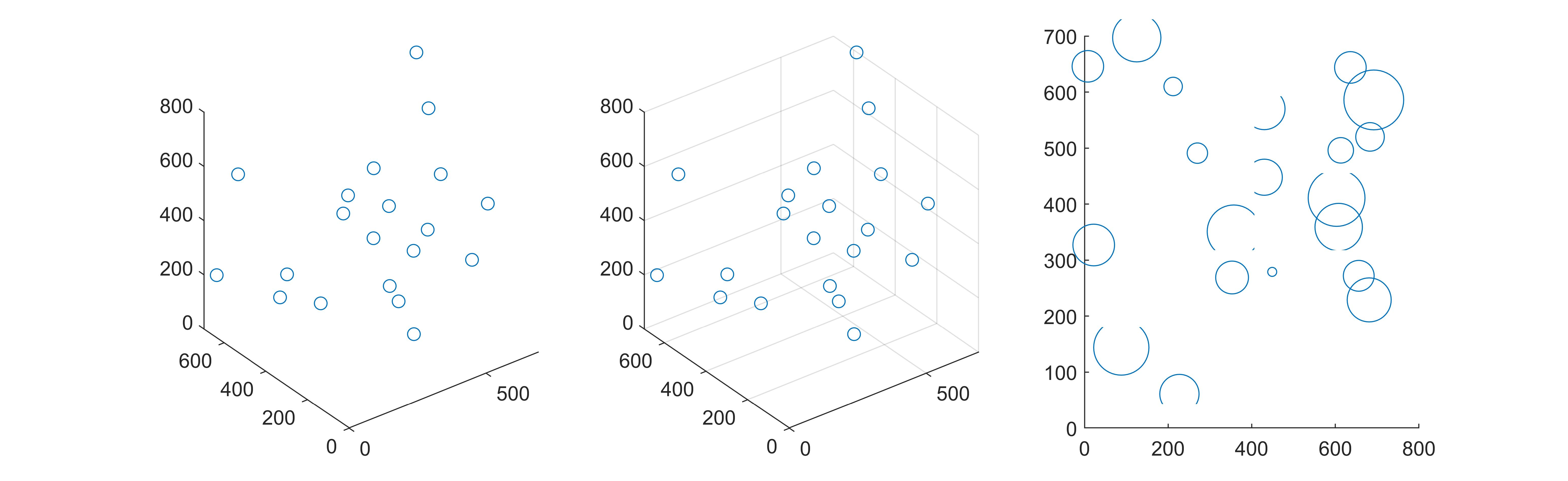• MATLAB生成随机点
千次阅读
2021-01-30 21:02:54

# MATLAB生成随机点

clc, close all, clear all

sc = 1.3;%定义缩放系数
xy_tra = 3;%定义标签平移距离
r = 400; %定义圆的半径
num_pt = 5000;%定义数据点个数
randr = r * rand(num_pt, 1); %生成随机点
phi = (2 * pi - 0) * rand(num_pt, 1); %生成随机角度

x = randr .* cos(phi);%生成随机x点
y = randr .* sin(phi);%生成随机y点

%循环生成(x1,y1)标签
%for i = 1 : num_pt
% xy_label(i,1) = cellstr([’ (x’,num2str(i),’, y’, num2str(i),’)’]);
%end

%生成半径300圆的数据
xs = r * cos(0:0.01:2pi);
ys = r
sin(0:0.01:2*pi);

figure(‘Position’, [50 50 600 600])%开图，定义图的原点和大小
hold on %开启hold on
box on %打开图的边框
axis equal %设图的xy轴相等
plot(xs,ys,‘r–’)%红色虚线为半径300的圆
scatter(x,y,a) %打x y的数据点。a为数据点的大小
text(x+xy_tra,y+xy_tra2,xy_label), box on, axis equal%打x y的标签
xlim([-sc
r, sc*r])%定义x轴范围
ylim = xlim; %设y轴范围和x轴范围相等

更多相关内容
• %生成均匀分布的随机整数，max生成随机整数最大值，生成m行n列的矩阵 编写函数sampling function [x,y,z]=sampling(lowx,upx,lowy,upy,lowz,upz,m,n) %该函数用来产生不重复的随机整数矩阵 %low—随机整数下界；...

randi函数

randi(max,m,n); %生成均匀分布的随机整数，max生成的随机整数最大值，生成m行n列的矩阵


编写函数sampling

function [x,y,z]=sampling(lowx,upx,lowy,upy,lowz,upz,m,n)
%该函数用来产生不重复的随机整数矩阵
%low—随机整数下界；up—随机整数上界；m—坐标点个数；n—各个坐标点之间的最小距离

%编写函数时的测试数据
% lowx=0;upx=700; lowy=0;upy=700; lowz=0;upz=800;m=20;n=10;

s=[];%矩阵s，第一行存储x，第二行存储y，第三行存储z
i=1;
c=1; %控制循环次数
while c<5000
tempx=randi([lowx,upx],1);
tempy=randi([lowy,upy],1);
tempz=randi([lowz,upz],1);
%如果矩阵s为空矩阵，则存储第一个随机数值
if(isempty(s)==1)
s(1,i)=tempx;
s(2,i)=tempy;
s(3,i)=tempz;
i=i+1;
c=c+1;
end
%如果矩阵s不为空矩阵，求出各个坐标之间的距离矩阵l，将与所有点之间距离都大于10的坐标存储
if(isempty(s)==0)
for j=1:i-1
l(1,j)=sqrt(((tempx-s(1,j))^2)+((tempy-s(2,j))^2)+((tempz-s(3,j))^2));
j=j+1;
end
if(isempty(find(l<n,1))==1)  %或size(find(l<n),1)==0 表示矩阵l中小于n的个数等于0
s(1,i)=tempx;
s(2,i)=tempy;
s(3,i)=tempz;
i=i+1;
end
c=c+1;
end
if(i>=m+1)
break;
end
end
x=s(1,:);
y=s(2,:);
z=s(3,:);


调用函数并绘制三维散点图

[x,y,z]=sampling(0,700,0,700,0,800,20,10);
subplot(131);plot3(x,y,z,'o');
subplot(132);scatter3(x,y,z,'o');
subplot(133);scatter(x,y,z,'o'); %二维展开全文• % 从当前轴得到N个，返回X坐标轴数据和Y轴坐标轴数据，用向量X和Y表示，长度为N。使用鼠标点击取。 % 按return健结束取。 % % [X,Y] = GINPUT gathers an unlimited number of points until the % return...

GINPUT Graphical input from mouse.

%   [X,Y] = GINPUT(N) gets N points from the current axes and returns

%   the X- and Y-coordinates in length N vectors X and Y.  The cursor

%   can be positioned using a mouse.  Data points are entered by pressing

%   a mouse button or any key on the keyboard except carriage return,

%   which terminates the input before N points are entered.

%   从当前轴得到N个点，返回X坐标轴数据和Y轴坐标轴数据，用向量X和Y表示，长度为N。使用鼠标点击取点。

%   按return健结束取点。

%

%   [X,Y] = GINPUT gathers an unlimited number of points until the

%   return key is pressed.得到无穷多个数据点，按回车键return时结束取点。

%

%   [X,Y,BUTTON] = GINPUT(N) returns a third result, BUTTON, that

%   contains a vector of integers specifying which mouse button was

%   used (1,2,3 from left) or ASCII numbers if a key on the keyboard

%   was used.

%

%   Examples:

%       [x,y] = ginput;

%

%       [x,y] = ginput(5);

%

%       [x, y, button] = ginput(1);

展开全文• Matlab针对图片处理的随机二维坐标生成 今天遇到一个问题，就是想要把图片处理成散点的方式，用opencv什么的不懂，也不是生成3D点云，就是简单的散点，就想通过Matlab的随机数的方式来生成，结果找了半天也没有合适...

# Matlab针对图片处理的随机二维坐标生成

今天遇到一个问题，就是想要把图片处理成散点的方式，用opencv什么的不懂，也不是生成3D点云，就是简单的散点，就想通过Matlab的随机数的方式来生成，结果找了半天也没有合适的代码。后来想了想，觉着自己很蠢，可以不局限于图片，可以先根据图片的像素点来生成多个随机数，而后将随机数用到图片里面，来去掉图片中的数值，也就是颜色。
代码如下：

a=randperm(17028,12000);

%imshow(I);
gray=double(rgb2gray(I))
[w,h]=size(gray);
gray(a)=0;
imshow(gray);

R=I(:,:,1); %red
G=I(:,:,2); %green
B=I(:,:,3); %blue
R(a)=0;
G(a)=0;
B(a)=0;
%imshow(R);
for i=1:w
for j=1:h
blue(i,j,1) = R(i,j);
blue(i,j,2) = G(i,j);
blue(i,j,3) = B(i,j);
end
end
imshow(blue,'border','tight','initialMagnification','fit');



效果是这样的：就先这样吧，肯定还有更好的方法，希望大神指教

展开全文• //////////////////////////////// matlab三维点坐标生成点云ply
• function p=createSimplyPoly(p) cen=mean(p); ang=atan2(p(:,1)-cen(1),p(:,2)-cen(2)); %每个坐标中心极角 p=[p,ang]; p=sortrows(p,3); %按极角排序 p=p(:,1:2); end
• 您好我想问一个问题如何在matlab中制作一个圆圈并标记其中心并在其中生成一定数量的随机点,例如50？我知道这个代码来制作一个圆圈x = linspace(-sqrt(10),sqrt(10));y1 = sqrt(10-x.^2);y2 = -sqrt(10-x.^2);plot(x,...
• 如何用MATLAB在指定范围内生成多个互不重叠的二维随机坐标 笔者做实验仿真时遇到了这个问题...当坐标点的可选范围足够小时，随机取点容易出现重叠现象，重点就在于“互不重叠”，对于二维坐标，互不重叠就意味着...
• 在半径为300和半径为50的同心圆的未相交部分随机生成N个 function [] = pos_generate(N, R, r, filename, seed) % R=300; r=50; % N: 生成N个, filename： 保存文件 % seed：随机种子 rng(seed); P=r+(R-r)*...python 矩阵
• 正如你所看到的,这只是几千的渐近(我运行了一个稍快的算法,反对200k来产生这个)：我不知道你是否曾尝试计算你的球体中可以适合的理论点数,但是我已经开始怀疑这个数字比1E6小得多.我用来研...
• 这里的另一种选择：%// Set parametersR = 0.5; %// radiusC = [3 4]; %// center [x y]N = 50; %// number of points inside circle%// generate circle boundaryt = linspace(0, 2*pi, 100);x = R*cos(t) + C(1);...
• 1.生成随机点： 主程序： clear; clc; picture_name = 1; %用来标记生成图片的序号 for i = 1 : 2 %要生成几张图片，就改成几次循环，这里是生成两张图片 RGB_set('R',2,12,'w'); %[背景色],[生成点个数]，[的...
• 有一个需求需要要生成地貌一样的随机地形图，首先我通过在固定大小的矩形面板上生成多个大小不一的矩形，然后赋予每个矩形随机的高度，然后再进行滤波得到想要的地形图。 上一篇已经生成随机的大小的矩形图，那么...随机数 矩形
• %覆盖区域：半径为r的圆 %输入参数--密度：lamda；半径：r 在半径为r的圆内用HPPP过程生成点坐标
• 本篇文章主要讲解在matlab环境下，如何随机生成满足高斯分布的样本。  众所周知，高斯分布是一种很常见的数据分布，也是很多实际例子中出现最多的一种分布。我们今天就来学习在matlab环境下绘制出随机分布的满足...高斯分布
• 对于矩形是随机生成两个数，然后在二维坐标系中组合成为四个；三角形状是随机生成三个坐标；至于圆原理类似；文字只是把原来要显示的形状变为字符串进行随机性输出。 而这里所说的随机性，主要有位置、大小和颜色...OpenCV rand
• (1条消息) 使用MATLAB在给定矩形区域随机绘制任意个不相交的圆_风一样的航哥的博客-CSDN博客 以上博客自从发表以来好几个同学都问我，三维的...% 此函数用来会绘制 N 个矩形区域里面随机生成的半径为 R 的圆，三维区..
• 这是一系列的运动坐标，第一次在(-3.5355,0,496.4645)处，下一次就到了(-8.5355,0,496.4645)处，以下就依次类推。我想问下怎么根据在这些不同的坐标连接成线画出来。每两个依次连接成直线，我只需要最后的三维...
• 简单写一个随机在一个三维区域生成球的matlab函数_风一样的航哥的博客-CSDN博客_comsol随机生成球体 上次简单写的功能，可以实现一些比较稀疏的分布，当分布比较密集的时候，会存在计算时间过长的问题，针对这个...
• 这将创建一组由笛卡尔坐标定义的随机点，并均匀分布在以原点为中心、半径为 r 的 n 维超球面的内部。 'randn' 函数首先用于创建 n 个随机变量的独立多元正态分布集，每个变量代表 n 维空间中的。 然后使用不完整...
• 一、matlab内置的密度函数于随机数生成器 离散均匀分布 离散均匀分布用于描述等概率发生事件的状况，仅限于有限的事件数 matlab提供{1，2，…，N}上的均匀分布的概率密度函数黑累计分布函数，其相应的命令为： ...开发语言
• matlab生成二维随机数，生成均值向量，协方差
• 程序在提供的 x 坐标点 P（输入）和提供的 256 位随机生成的私钥 d（输入）上执行标量乘法。 程序默认为点 (x=9)，它是曲线 25519 的基点。 输入应为十六进制（小端）。 程序仅使用各种私钥在几个点上进行了测试。 ...
• 输入文件示例是mag_out.txt，由包含3个数字的行组成，这些数字代表点坐标。 使用plot_ellipsoid.py查看输入数据，拟合的椭球以及将数据传输到球体表面上。 使用get_calibration_ellipsoid.py生成magcal_ellipsoid....
• %提取非生长核边界格索引 En=rand(1,length(CInE));%各个单元均会被赋值一个随机数 [i,j]=find(En);%大于该方向上的方向增长概率数，则此单元同样成为一个新的生长相 pE=sub2ind([1,length(CInE)],i,j);%新的生长...
• (1)生成正样本%% 读取文件夹是需要提取样本的视频采集图片，里面的1.txt是人工标出的目标坐标%格式如下：img_1.jpg 2 4 527 215 524 238 535 238 537 215 4 575 218 571 238 581 238 580 218img_2.jpg 2 4 535 217 ...
• 案例基本情况：10*10区域，随机均布50*50=2500个粒子，坐标随机产生，颗粒为4种类型，大小分为5种，然后用matlab编制小程序进行图形绘制。 主要程序如下： clc;clear;close all; Nx=50; Ny=50; N=Nx*Ny; %的...算法
• 这组随机点总共有十个，通过rand()函数在0-1这个范围内分别获取了十个x坐标和十个y坐标，组成了十个坐标点，这十个坐标点随机的。 2.将这一组坐标点分成两组，并用不同的颜色区分 x=rand(1,10); y=rand(1,10); x1=......

# matlab随机生成坐标点matlab 订阅